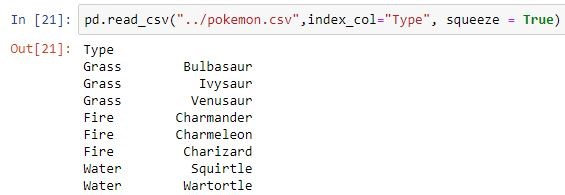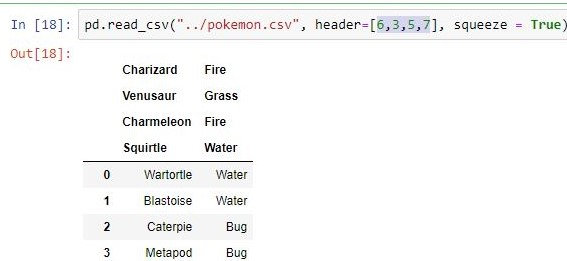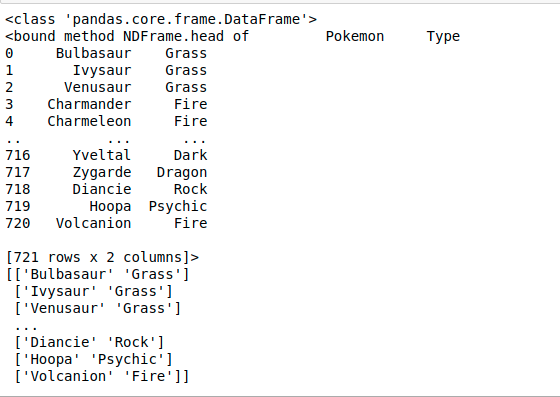# Python program to read CSV without CSV module

• Last Updated : 30 Jan, 2020

CSV (Comma Separated Values) is a simple file format used to store tabular data, such as a spreadsheet or database. CSV file stores tabular data (numbers and text) in plain text. Each line of the file is a data record. Each record consists of one or more fields, separated by commas. The use of the comma as a field separator is the source of the name for this file format.

CSV files can be read using the Python library called `Pandas`. This library can be used to read several types of files, including CSV files. We use the library function read_csv(input) to read the CSV file. The URL/path of the CSV file which you want to read is given as the input to the function.

Syntax:

pd.read_csv(filepath_or_buffer, sep=’, ‘, delimiter=None, header=’infer’, names=None, index_col=None, usecols=None, squeeze=False, prefix=None, mangle_dupe_cols=True, dtype=None, engine=None, converters=None, true_values=None, false_values=None, skipinitialspace=False, skiprows=None, nrows=None, na_values=None, keep_default_na=True, na_filter=True, verbose=False, skip_blank_lines=True, parse_dates=False, infer_datetime_format=False, keep_date_col=False, date_parser=None, dayfirst=False, iterator=False, chunksize=None, compression=’infer’, thousands=None, decimal=b’.’, lineterminator=None, quotechar='”‘, quoting=0, escapechar=None, comment=None, encoding=None, dialect=None, tupleize_cols=None, error_bad_lines=True, warn_bad_lines=True, skipfooter=0, doublequote=True, delim_whitespace=False, low_memory=True, memory_map=False, float_precision=None)

Not all of them are much important but remembering these actually save time of performing same functions on own. One can see parameters of any function by pressing shift + tab in jupyter notebook. Useful ones are given below with their usage :

Parameter Use
filepath_or_buffer URL or Dir location of file
sep Stands for seperator, default is ‘, ‘ as in csv(comma seperated values)
index_col Makes passed column as index instead of 0, 1, 2, 3…ruse_cols Only uses the passed col[string list] to make data frame
squeeze If true and only one column is passed, returns pandas series
skiprows Skips passed rows in new data frame

If the given path is invalid ie the file is not present at the given path then the function gives a `FileNotFoundError`. But if the function successfully reads the file, then it returns an object of type `class pandas.core.frame.DataFrame`. The returned dataframe(Object) can then be converted to a numpy array by using the function `dataframe.to_numpy()` this function comes with pandas and returns the numpy array representation of the dataframe. Then onwards we can use `arr` as a numpy array to perform desired operations.

Example:

 `# PYthon program to read  ` `# CSV file without csv module ` ` `  ` `  `import` `pandas as pd ` ` `  ` `  `#reading a csv file with pandas ` `data_frame ``=` `pd.read_csv(``"pokemon.csv"``)    ` `  `  `#give the datatype of a pandas  ` `# object ` `print``(``type``(data_frame))   ` `  `  `#this function gives us a  ` `# brief view of the data. ` `print``(data_frame.head) ` `  `  `#converting pandas dataframe ` `# to a numpy array. ` `arr ``=` `data_frame.to_numpy() ` `print``(arr) `

Output:My Personal Notes arrow_drop_up
Recommended Articles
Page :